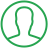# How To Reverse A String In Python

Last Updated : Oct 6, 2023In this article we will show you the solution of how to reverse a string in python, a common programming procedure that is needed in many applications is reversing a string. Using a stack data structure is one of the easiest and most natural ways to reverse a string in Python.

An element can be added to or removed from the top of a stack of elements using the push and pop procedures, respectively.

The reversed string can be created by stacking each character in the string, then popping them off in the opposite sequence.

A string can also be reversed using built-in Python functions and techniques like join(), slice(), and reversed().

The idea of reversing a string in Python will now be discussed.

## Step By Step Guide On How To Reverse A String In Python :-

```def createStack():
stack = []
return stack
def size(stack):
return len(stack)
def isEmpty(stack):
if size(stack) == 0:
return true
def push(stack, item):
stack.append(item)
def pop(stack):
if isEmpty(stack):
return
return stack.pop()
def reverse(string):
n = len(string)
stack = createStack()
for i in range(0, n, 1):
push(stack, string[i])
string = ""
for i in range(0, n, 1):
string += pop(stack)
return string
s = "Talkerscode"
print("The Given string is : ", end="")
print(s)
print("Result : ", end="")
print(reverse(s))```
1. You can see in this example how we wrote Python code to reverse a string.
2. The function createStack(), which produces an empty stack & returns it, is used at the beginning of the code.
3. When a stack is used as a parameter, the size() function uses the len() function to return the number of elements in the stack.
4. The isEmpty() function then calls size() to see whether the stack is empty or not and returns True if the size is zero.
5. Then, using the append() method, the push() function adds an item to the top of the stack by taking a stack and an item as arguments.
6. Then, using the pop() method, the pop() function takes the stack as an argument, removes the top element from the stack, and then returns it.
7. The function returns None if there is no data on the stack.
8. After that, the reverse() method accepts a string as an argument, uses createStack() to build an empty stack, and then uses a for loop to go through each character in the string.
9. It uses push() to add each character to the stack.
10. Once the loop is finished, another for loop is used to iterate through the stack while creating an empty string.
11. It uses pop() to remove each piece from the stack before appending it to the empty string.
12. It then gives back the reversed string.
13. Next, a string named "Talkerscode" is defined.
14. It uses the print() method to print the original string before invoking reverse() with the argument s.
15. The reversed string is then printed.

## Conclusion ;-

As a result, we have successfully understood the concept of string reversal in Python.

Python also showed us how to reverse a string in an easy and effective manner.

Python has built-in functions and methods like join(), slice(), and reversed() that can also be used for this purpose. However, using a stack is a natural way to reverse a string.

I hope this article on how to reverse a string in python helps you and the steps and method mentioned above are easy to follow and implement.

##About Amruta

Amruta is an Experienced web developer with 4 years for experience she completed her master's with MCA and passionate about programming Languages for creating technical contents like HTML, CSS, JavaScript, Java, Python, PHP, jQuery.